Номер части: Журнал
ISSN: 2411-6467 (Print)
ISSN: 2413-9335 (Online)
Статьи, опубликованные в журнале, представляется читателям на условиях свободной лицензии CC BY-ND

# Determination of energy gaps of transistors and diodes by simple electric circuit

Науки и перечень статей вошедших в журнал:
DOI:
Дата публикации статьи в журнале:
Название журнала: Евразийский Союз Ученых — публикация научных статей в ежемесячном научном журнале, Выпуск: , Том: , Страницы в выпуске: -
Данные для цитирования: . Determination of energy gaps of transistors and diodes by simple electric circuit // Евразийский Союз Ученых — публикация научных статей в ежемесячном научном журнале. Физико-математические науки. ; ():-.

Introduction

The real start of the atomic structure takes place in 1900 by the discovery of Max Plank that light can not treated as wave but as a discrete quantum of energy called photons .The photon concept can describe  some physical phenomena like black body radiation, photoelectric effect, beside atomic spectroscopy. The atomic spectroscopy is associated with the discovery that each atom emits electromagnetic wave having only certain specific frequencies. These frequencies and wavelengths are discovered by using spectrometers .

People tried to explain the atomic spectra by suggesting certain atomic models. The most popular model is that suggested by Bohr in 1913. In his models Bohr suggested that each atom consists at a nucleus around which electrons revolves in certain orbits.

When an electron moves from a higher orbit to a lower one, the atom emits a photon of energy equal to the difference between the energy of the two orbits .

Although Bohr model for the atom agrees well with experimental spectroscopy, but it does not rest on a solid theoretical ground.

This motivates Heisenberg in 1925 and Schrödinger in 1926 to formulate a full quantum theory that rests on a solid theoretical ground and can explain most of atomic phenomena .

Most of experimental works in quantum mechanics is based on the atomic spectra, which is widely used in many applications. The importance of spectroscopy make it very important for the physicists to do intensive experimental work in this field. Unfortunately, the experiments needs sophisticated and expensive devices, which are rare in third world countries. Thus, one needs to select a path way to solve this problem.

The aim of the work is to design a simple electrical circuit to find the energy gaps for bulk materials, namely semiconductors. The effect of temperature and a magnetic field on the energy band is also investigated.

Different a attempts were made to determine energy levels and bands, like Frank Hertz experiments . The magnetic field and temperature effect of energy levels and bands as shown by some researches .

In solid-state physics, a band gap, also called an energy gap or band gap, is an energy range in a solid where no electron states exist. In a graph of the electronic band structure of a solid, the band gap generally refers to the energy difference  between the top of the valence band and the bottom of the conduction band which is found in insulators and semiconductors. It is the amount of energy required to free an electron from the top of the valence band to the bottom of the conduction band    to become a mobile charge carrier, able to move freely within the solid material. In conductors, the two bands often overlap, so they may not have a band gap . In semiconductors and insulators, electrons are confined to a number of bands of energy, and forbidden from other regions. The term «band gap» refers to the energy difference between the top of the valence band and the bottom of the conduction band; electrons are able to jump from one band to another. In order for an electron to jump from a valence band to a conduction band, it requires a specific minimum amount of energy for the transition. The required energy differs with different materials. Electrons can gain enough energy to jump to the conduction band by absorbing either a phonon (heat) or a photon (light) . A material with a small but nonzero band gap which behaves as an insulator at absolute zero but allows thermal excitation of electrons into its conduction band at temperatures which are below its melting point is referred to as a semiconductor. A material with a large band gap is called an insulator. In conductors, the valence and conduction bands may overlap, so they may not have a band gap .

The conductivity of intrinsic semiconductors is strongly dependent on the band gap. The only available carriers for conduction are the electrons which have enough thermal energy to be excited across the band gap.Band gap engineering is the process of controlling or altering the band gap of a material by controlling the composition of certain semiconductor alloys, such as GaAlAs, InGaAs, and InAlAs. It is also possible to construct layered materials with alternating compositions by techniques like molecular beam epitaxy. These methods are exploited in the design of heterojunction bipolar transistors (HBTs), laser diodes and solar cells .

The distinction between semiconductors and insulators is a matter of convention. One approach is to think of semiconductors as a type of insulator with a narrow band gap. Insulators with a larger band gap, usually greater than 3 eV, are not considered semiconductors and generally do not exhibit semiconductive behaviour under practical conditions. Electron mobility also plays a role in determining a material’s informal classification .The band gap energy of semiconductors tends to decrease with increasing temperature. When temperature increases, the amplitude of atomic vibrations increase, leading to larger inter atomic spacing. The interaction between the lattice phonons and the free electrons and holes will also affect the band gap to be smaller .

Experimental setup and sample preparation:

The circuit, which is designed to find the energy levels and gaps of some semiconductor devices, is presented. The samples, which are selected beside the experimental procedures, are also discussed. The aim of this work is to use a simple electric circuit to find the energy gaps of some semiconductors. This can be done by relating current to voltage and see the position where the current changes abruptly these position are related to the energy gaps.

Samples:

The samples, which are utilized, are electronic components, which are made from semiconductors, the samples are as follows: Two light emitting diodes (LED), three ordinary Diodes, two light Diode resistors (LDR), two Transistors Bipolar Junction transistors (BJT).

Circuit Design:

The circuit is designed to find the energy gap about 100 readings for voltage and current in the voltage in the range of milli volt were made.

The circuit consists of a power supply having voltage range in milli volts (mV). The semiconductor device is connected in series with a meter of current range of mA. This semiconductor is connected in parallel with a voltammeter having arange of milli (mV). To see how heat and magnetic field affect the energy band the semiconductors are exposed to heat and magnetic field of intensities (4250, 3080, 2500, 1740 milli Tesla).

Experimental procedures:

The following steps to find the energy band gap should be done:

• Each semiconductor electronic component is connected.
• The power supply voltage in increased gradually in steps of milli volts (mV). The volt and the corresponding current is recorded. The readings are done 100 times.
• The relation between V and I for each sample is drawn graphically.
• The effect of heat on the semiconductor is determined by exposing the semiconductor to temperatures 15, 18, 21…..in steps 30 C up to 450
• The effect of the magnetic field is determined by exposing the semiconductor to magnetic field of intensity (4250, 3080, 2500, 1740 milli Tesla) at different distances.
• The volts at which the current drops or rise abruptly is recorded. The energy gap Eg is thus given by the energy difference between two successive points of V.

The Results:

A light emitting diode (LED) is connected with the circuit shown in figure (a) Fourty five readings for V and I were obtained .For one light emitting diode as  a sample .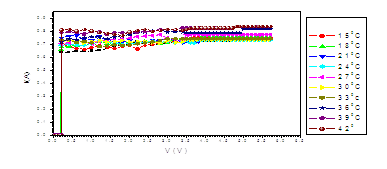Figure (a). V versus I for LED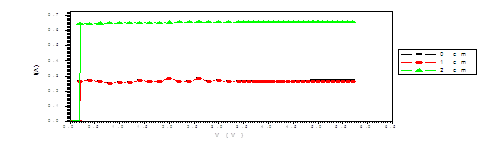Figure (b). V versus I for LED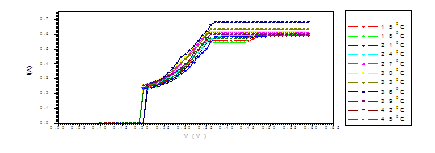Figure (c). V versus I for LED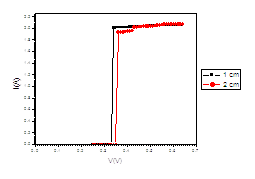Figure (d). V versus I for LED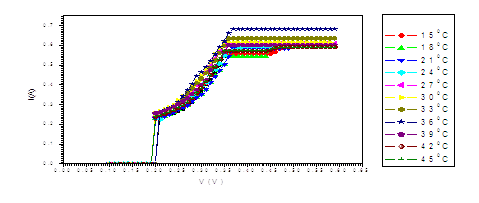Figure (e). V versus I for LED at different temperatures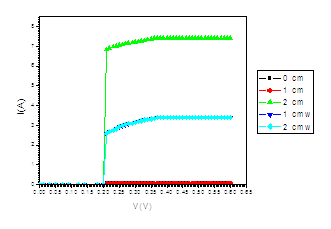Figure (f). V versus I for LED at different distances under the effect of magnetic field of intensity (4250, 3080, 2500, 1740 mT)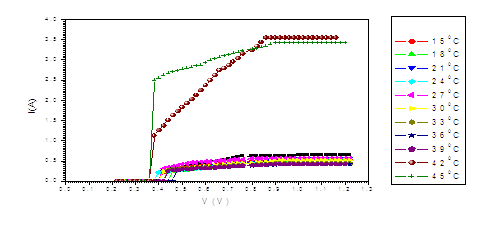Figure (g). V versus I for Transistor at different temperatures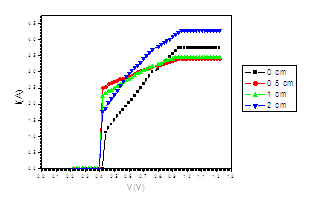Figure (h). V versus I Transistor at different distances under the effect of magnetic field of intensity (4250, 3080, 2500, 1740 mT)

The Results of energy gap:

Light Emitting Diode

a- for different temperatures.               b- At different distances for magnetic field diode

 Degree/0C Eg/eV distance/Cm Eg/eV 15 0.1 0 0.2 18 0.1 1 0.2 21 0.1 2 0.19 24 0.1 27 0.1 30 0.1 33 0.1 36 0.1 39 0.1 42 0.1 45 0.1

a- for different temperatures.               b- At different distances for magnetic field LDR

 Degree/0C Eg/eV distance/Cm Eg/eV 15 0.2 0 0.2 18 0.21 1 0.33 21 0.21 2 0.34 24 0.2 27 0.2 30 0.2 33 0.22 36 0.22 39 0.22 42 0.2 45 0.2

a- for different temperatures.        b- At different distances for magnetic field Transistor

 Degree/0C Eg/eV distance/Cm Eg/eV 15 0.20 1 0.2 18 0.2 2 0.2 21 0.2 0 Without light 0.21 24 0.2 1 Without light 0.23 27 0.2 30 0.2 33 0.2 36 0.22 39 0.21 42 0.21 45 0.2

a- for different temperatures.        b- At different distances for magnetic field Discussion

 Degree/0C Eg/eV distance/Cm Eg/eV 15 0.46 0 0.41 18 0.42 0.5 0.4 21 0.4 1 0.39 24 0.4 2 0.38 27 0.42 30 0.42 33 0.41 36 0.41 39 0.41 42 0.41 45 0.4

The figure (a) for LED shows that the current increases abruptly at 0.2 eV. The temperature does not affect the energy gap of current. But figure (b) shows the abrupt current decrease of about 0.4 milli A, which may result from the broadening of the gap by the magnetic field. Figure (c) for Diode shows abrupt increase at about 0.2 eV. The magnetic field effects shows abrupt change for diode in figure (d) at about 0.34 eV. Thus, the magnetic field broadens the energy gap. For LDR in figure (e) the gap is about 0.2 eV while in figure (f) the abrupt change is about 0.23 eV, again the magnetic field broadens the gap. Transistors in figure (g) shows that the current increases abruptly at 0.4 eV, while for figure (h) are about 0.42 eV for transistors under the effect of magnetic field. This may be explained on the basis of bands theory. The current increases abruptly when the voltage energy supplied to the electron just equals or exceeds slightly the energy gap. In this case large number of electrons cross to the conduction band. Thus increasing the current abruptly according to the relation:

Where any increase in free carries concentration increases the current. Sometimes the current remains almost constant when the voltage is increased. This may be attributed to the fact that the energy gained by electrons to jump from the valence to the conduction band through the energy gap is less than the value of the energy gap Eg, I.e.

The values at which the current increases abruptly Show that the energy gaps for LED are in the range of (0.2-0.25) eV, these values are lower than the typical values (0.24-3.03). For the ordinary diodes the energy gaps are in the range (0.2 – 0.34) eV, which again are less than the typical values (0.24-3.03). For the LDR the energy gaps are in the range (0.2- 0.23) eV, which again are less than the typical values (0.24-3.03).The same hold for the transistors which show that the Eg are in the range (0.4 – 0.42) eV.

The low values for the energy gap may result from the heavy doping which causes the energy gap to be narrow. The energy levels of the impurities usually stretches them selves with in the energy gap. The results obtained may suggest that at room temperature thermal energy causes electrons to leave conduction band to impurity levels. Then when the voltage is applied electrons jump in the conduction band form impurity levels. The ranges of the energy gap 0.2eV suggests that the sample may be doped by Cu as a doner, while the energy gap 0.4eV indicates doping with Fe donors .

Conclusion:

This work shows that simple electric circuits can be utilized to find the energy gaps and levels for semiconductors. This requires to take a bout 50 or more readings for I and V.

The position where the current remains almost constant or increases abruptly can be used to find   the values of the energy gap.

References:

1. Demystified David Mc Mahon, 1999,Quantum Mechanics, Mc Graw-Hill, Newyork, PP.393 .
2. WWW [https://webelements.com/]Mark Winter [The University of Sheffield and WebElements Ltd, UK,2010.
3. J. Burns ,1985, Solid State Physics (Academic press, Florida),PP. 420.
4. B.H. Bransden & C.J. Joachain, 2000, Quantum Chemistry of solid ,McGraw-Hill, Oxford University Press, ,PP.883.
5. David J. Griffiths. 2003, Introduction of Quantum Mechanics ,Prentice Hall, U.S.A , PP. 394.
6. Charles Kittel, 2003, Introduction Of Solid State Physics, PP. 704 .
7. S.O. kasap, 2000, Principles Of Electronic Materials and Devises, University of Cambridge, England, electrical Engeering university of Saskat chewan Canada, PP. 232.
8. Charles M. wolfe, Nick Holon yak, JR, Gregory, E. Stillman, 2001, Physical Properties Of Semiconductors, Prentice Hall, Engle wood-Cliffs, PP. 354.
9. Sakurai, J. 1985, Modern Quantum Mechanic, Addision- Wesley, (U.S.A,Oklahoma), PP. 500.
10. J.J. Sakurai, 1994, Modern Quantum Mechanic, Addison- Wesley pub.Co.Reading , PP. 500.
11. D.J.Griffiths,1995, introduction Quantum Mechanic, prentice Hall, engle wood Cliffs, PP. 384.
12. Donald A. Neamen, 1997, Semiconductor Physics & Devices, Mc Graw-Hill, University of newmaxico, PP. 746.
13. P.S. Kireev, 1975, Semiconductor Physics , First published,(Mir,Moscow), PP. 118.
14. Hiat. A.M. Salih, 2009, Determination of energy gaps and levels of ordinary and light emitting diodes by an electrical method ,M.Sc. Alzaeem Alazhari University, Khartoum.[schema type=»book» name=»Determination of energy gaps of transistors and diodes by simple electric circuit» description=»In this work, two light emitting diodes, two ordinary diodes, two light dependent resistors, beside two transistors were connected to a simple electric circuit. The circuit comprised of a power supply, an ammeter and a voltmeter. Fifty (50) readings for voltage (mV) and current (mA) were recorded. A graph relating the current to the voltage was drawn for only one sample from each semiconductor type. It was found that for light emitting diode, ordinary diode, light dependent resistor the current increased abruptly at about 0.2 Volt, where as it changes abruptly at about 0.4 Volt for transistors. The results indicated the poribility of the existence of energy gaps. The value of the corresponding gaps were less than the ordinary values, which may result from the fact that the doping levels exist inside the energy gaps. Exposure of the sample to the heat increased the current which is consistant with the fact that heat increases electron velocity. The exposure of samples to the magnetic field broadens the energy gap which is in agreement with previous reports.» author=»Hamad, Kamal Ali» publisher=»БАСАРАНОВИЧ ЕКАТЕРИНА» pubdate=»2017-02-07″ edition=»ЕВРАЗИЙСКИЙ СОЮЗ УЧЕНЫХ_29.08.15_08(17)» ebook=»yes» ]
Список литературы:

##### euroasia
Записи созданы 9819

## РЕШЕНИЕ ЗАДАЧИ ФИЛЬТРАЦИОННОЙ КОНСОЛИДАЦИИ ГРУНТА С УЧЕТОМ ИЗМЕНЕНИЯ ТЕПЛОФИЗИЧЕСКИХ ХАРАКТЕРИСТИК

Начните вводить, то что вы ищите выше и нажмите кнопку Enter для поиска. Нажмите кнопку ESC для отмены.

Вернуться наверх